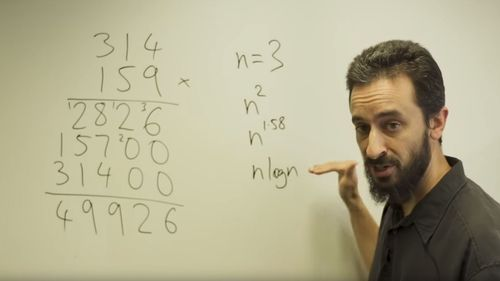PUBLISHED

# Newly Cracked Math Puzzle Allows Faster Multiplication Of Large NumbersA UNSW Sydney mathematician has cracked a maths problem that has stood for almost half a century. UNSW Science

Back in 1971, two German mathematicians speculated it would be possible to multiply large numbers (as in, veeery large numbers) using an incredible fast method, known as the Schönhage-Strassen algorithm. However, this bright idea remained hypothetical for several decades – until now.

Associate Professor David Harvey, a mathematician from the University of New South Wales (UNSW) in Australia, has solved the 48-year multiplication puzzle first proposed by Arnold Schönhage and Volker Strassen, a breakthrough which will allow computers to multiply large numbers with greater efficiency and speed.

To understand how this method works, take your mind back to the way you learned to multiply numbers in elementary school. If you wanted to multiply something like 159 x 314, for example, you would write the two numbers on top of each other, multiply every single digit of the number with each digit of the other number, then add up the new numbers. This method requires you to calculate 9 separate multiplication operations.

Since both the numbers in this multiplication have 3 digits (n = 3), each n digit of the first number has to be multiplied by each n digit of the second number, which is the equivalent to n2. In 1971, Schönhage and Strassen proposed it is theoretically possible to do this multiplication with far fewer operations, using just n * log(n) operations, but were unable to prove it at the time. The new work shows there is, indeed, an algorithm that does just this.

You can read the research paper written by Harvey and his collaborator Joris van der Hoeven from the École Polytechnique in France on the open access archive HAL. It's also explained rather nicely by Harvey himself in the video below.

“They predicted that there should exist an algorithm that multiplies n-digit numbers using essentially n * log(n) basic operations," Harvey said in a  "Our paper gives the first known example of an algorithm that achieves this.”

If a computer were to use the old “elementary school method” to multiply two numbers with billions of digits each, it would take months. However, it would take under 30 seconds using the Schönhage-Strassen algorithm.

Schönhage and Strassen also suggested that n * log(n) is the “best possible” result. In effect, this would be the fastest multiplication algorithm possible. While it would take a huge amount of work to ever prove this, it’s certainly a tantalizing idea.

So, this all sounds pretty impressive, but what's the real use of all this? By allowing faster multiplication, researchers could use it to calculate digits of pi more efficiently than before and solve problems involving huge prime numbers.

"People have been hunting for such an algorithm for almost 50 years. It was not a foregone conclusion that someone would eventually be successful," Harvey summed up. "It might have turned out that Schönhage and Strassen were wrong, and that no such algorithm is possible. But now we know better."

### ARTICLE POSTED IN

•• math,

• mathematics,

• maths,

• multiplication,

• multiply